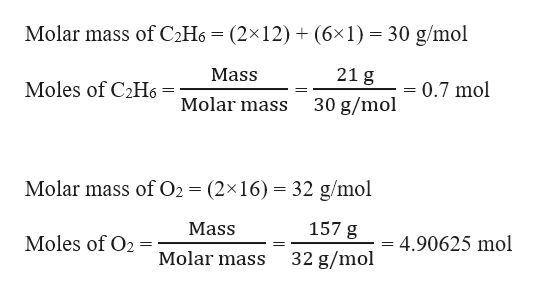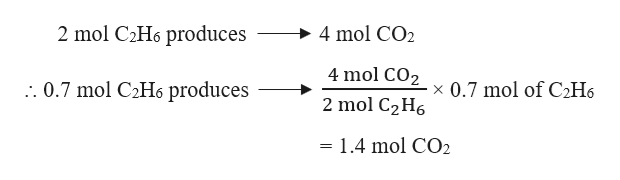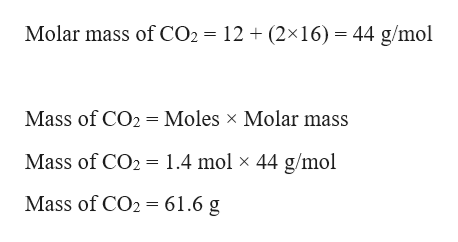# -2Sio Si Fa4mg 7g9.12:17 AM Thu Dec 5DoneDoneAAe www-awu.ale s.comO STOICHIOMETRYLimiting reactantsJaqueshaGaseous ethane (CH,CH,) will react with gaseous oxygen (02) to produce gaseous carbon dioxide (CO2) and gaseous water (H,0). Suppose 21. g ofethane is mixed with 157.g of oxygen. Calculate the maximum mass of carbon dioxide that could be produced by the chemical reaction. Be sure your answerhas the correct number of significant digits.x10

Question
20 views

How do I solve?help_outlineImage Transcriptionclose-2 Sio Si Fa 4 mg 7g 9. 12:17 AM Thu Dec 5 Done Done AA e www-awu.ale s.com O STOICHIOMETRY Limiting reactants Jaquesha Gaseous ethane (CH,CH,) will react with gaseous oxygen (02) to produce gaseous carbon dioxide (CO2) and gaseous water (H,0). Suppose 21. g of ethane is mixed with 157.g of oxygen. Calculate the maximum mass of carbon dioxide that could be produced by the chemical reaction. Be sure your answer has the correct number of significant digits. x10 fullscreen
check_circle

Step 1

Given,

Mass of ethane, (CH3CH3) C2H6 = 21 g

Mass of oxygen, O2 = 157 g

Moles of C2H6 and O2 can be calculated as:help_outlineImage TranscriptioncloseMolar mass of C2H6 = (2×12) + (6×1) = 30 g/mol 21 g Mass Moles of C2H6 = 0.7 mol 30 g/mol Molar mass Molar mass of O2 = (2×16) = 32 g/mol 157 g Mass Moles of O2 4.90625 mol 32 g/mol Molar mass fullscreen
Step 2

The balanced chemical reaction of gaseous ethane with oxygen to produce gaseous carbon dioxide and gaseous water can be written as:

2 C2H6 (g) + 7 O2 (g) → 4 CO2 (g) + 6 H2O (g)

Stoichiometric molar ratio of C2H6 : O2 = 2 : 7 = 1 : 3.5

Actual molar ratio of C2H6 : O2 = 0.7 : 4.90625 = 1 : 7

Therefore, O2 is present in excess and C2H6 is the limiting reagent. Therefore, moles of CO2 produced will depend on C2H6.

From the given reaction, it is evident that :help_outlineImage Transcriptionclose2 mol C2H6 produces 4 mol CO2 4 mol CO2 .. 0.7 mol C2H6 produces x 0.7 mol of C2H6 2 mol C2H6 = 1.4 mol CO2 fullscreen
Step 3

Mass of CO2 can be calcu...help_outlineImage TranscriptioncloseMolar mass of CO2 = 12 + (2×16) = 44 g/mol Mass of CO2 = Moles x Molar mass Mass of CO2 = 1.4 mol × 44 g/mol Mass of CO2 = 61.6 g fullscreen

### Want to see the full answer?

See Solution

#### Want to see this answer and more?

Solutions are written by subject experts who are available 24/7. Questions are typically answered within 1 hour.*

See Solution
*Response times may vary by subject and question.
Tagged in

### General Chemistry